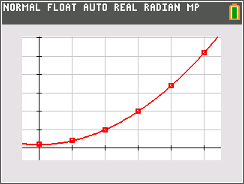### Count the Differences

Students are given data, asked to find the finite differences, and then use this to find a polynomial that models the data.
•TI-84 Plus CE
•TI-84 Plus C Silver Edition
• TI-84 Plus Silver Edition
• TI-84 Plus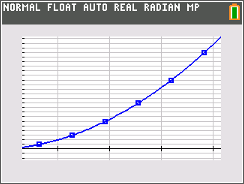### Stacking Bricks

This activity presents a real-world situation--stacking bricks in a pile--that can be modeled by a polynomial function. Students create a small table to show how the number of bricks relates to the number of rows, and calculate the first, second, and third differences of the data. Next they use the graphing calculator's statistical calculation functions to perform the correct regression. Finally, they evaluate the model using a variety of methods.
•TI-84 Plus CE
•TI-84 Plus C Silver Edition
• TI-84 Plus Silver Edition
• TI-84 Plus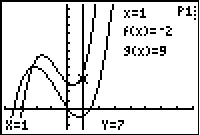### Building curves

Students approach performing the basic operations on the polynomials from a graphical perspective. Given the graphs of two functions, they plot points that lie on the graph of the sum of the functions and draw conclusions about its behavior. Next, they calculate a regression to fit the points they plotted. Finally, they find the sum of the functions algebraically and compare it with the result of the regression.
•TI-84 Plus CE
•TI-84 Plus C Silver Edition
• TI-84 Plus Silver Edition
• TI-84 Plus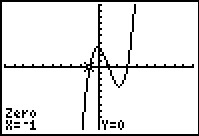### One of the Many Ways

Students graph polynomials to determine the value and number of zeros for a given polynomial.
•TI-84 Plus CE
•TI-84 Plus C Silver Edition
• TI-84 Plus Silver Edition
• TI-84 Plus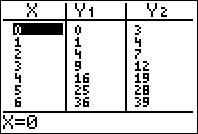### Transformers

Students explore the different transformations of several polynomial functions.
• TI-84 Plus Silver Edition
• TI-84 Plus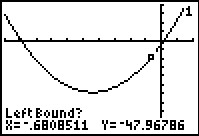••